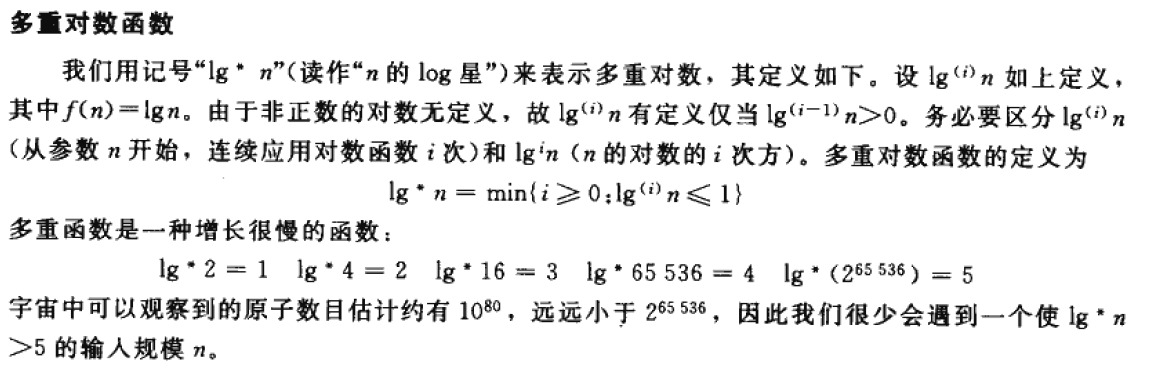# 多重对数函数The iterated logarithm function的定义

Functional iteration

We use the notation f(i)(n) to denote the function f(n) iteratively applied itimes toan initial

value of n. Formally, let f(n) be a function over the reals.For nonnegative integers i, we

recursively define

For example, if f(n) = 2n, then f(i)(n) = 2in.

The iterated logarithm function

We use the notation lg* n (read "log star of n") to denote the iteratedlogarithm, which is

defined as follows. Let lg(i) n be as defined above, with f(n) = lg n. Because the logarithm of a

nonpositive number is undefined, lg(i) n is defined only if lg(i-1)n > 0. Be sure to distinguish

lg(i) n (the logarithm function applieditimes insuccession, starting with argument n) from lgi

n (the logarithm of n raised to the ith power). The iteratedlogarithm function is defined as

lg* n= min {i= 0: lg(i) n 1}.

The iterated logarithm is a very slowly growing function:

lg* 2 = 1,

lg* 4 = 2,

lg* 16 = 3,

lg* 65536 = 4,

lg*(265536) = 5.

Since the number of atoms in the observable universe is estimated to beabout 1080, which is

much less than 265536, werarely encounter an input size n such that lg* n > 5.